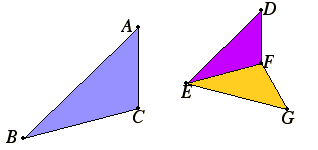# Proposition 5

If two triangles have their sides proportional, then the triangles are equiangular with the equal angles opposite the corresponding sides.

Let ABC and DEF be two triangles having their sides proportional, so that AB is to BC as DE is to EF, BC is to CA as EF is to FD, and further BA is to AC as ED is to DF.

I say that the triangle ABC is equiangular with the triangle DEF where the equal angles are opposite the corresponding sides, namely the angle ABC equals the angle DEF, the angle BCA equals the angle EFD, and the angle BAC equals the angle EDF.Construct the angle FEG equal to the angle CBA and the angle EFG equal to the angle BCA on the straight line EF and at the points E and F on it. Therefore the remaining angle at A equals the remaining angle at G.

Therefore the triangle ABC is equiangular with the triangle GEF.

VI.4

Therefore in the triangles ABC and GEF the sides about the equal angles are proportional where the corresponding sides are opposite the equal angles, therefore AB is to BC as GE is to EF.

V.11

But, by hypothesis, AB is to BC as DE to EF, therefore DE is to EF as GE is to EF.

V.9

Therefore each of the straight lines DE and GE has the same ratio to EF, therefore DE equals GE.

For the same reason DF also equals GF.

Then since DE equals GE, and EF is common, the two sides DE and EF equal the two sides GE and EF, and the base DF equals the base GF, therefore the angle DEF equals the angle GEF, and the triangle DEF equals the triangle GEF, and the remaining angles equal the remaining angles, namely those opposite the equal sides.

Therefore the angle DFE also equals the angle GFE, and the angle EDF equals the angle EGF.

And, since the angle DEF equals the angle GEF, and the angle GEF equals the angle ABC, therefore the angle ABC also equals the angle DEF.

For the same reason the angle ACB also equals the angle DFE, and further, the angle at A equals the angle at D, therefore the triangle ABC is equiangular with the triangle DEF.

Therefore, if two triangles have their sides proportional, then the triangles are equiangular with the equal angles opposite the corresponding sides.

Q.E.D.

## Guide

Of course, this proposition is the converse of the previous. We now have two characterizations of similar triangles, either as equiangular triangles or as triangles with proportional sides. The next two propositions give two more characterizations corresponding to characterizations of congruent triangles.

As in VI.2, a certain order is assumed for the proportionality. It is not intended, for instance, that AB : BC = DE : EF while BC : CA = FD : EF. See the remark about VI.Def.1.

This proposition is used in the proof of proposition XII.12.